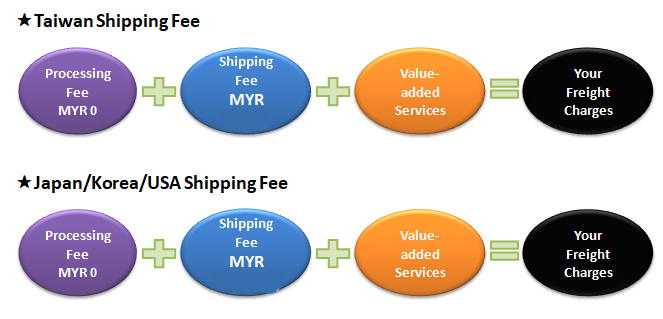Home > Rates & Fees > Freight Weight CalculationFreight weight is calculated based on Volumetric Weight of Length (cm) x Width (cm) x Height (cm) / 6000.
Minimum weight of 3.0kg imposed for South Korea until further notice.

Example:
1.2kg (round up to 2.0kg) + 1.3kg (round up to 2.0kg) + 0.7kg (round up to 1.0kg) = 5.0kg
Chargeable freight weight is round to the nearest kilogram per parcel, final calculated weight is 5kg.